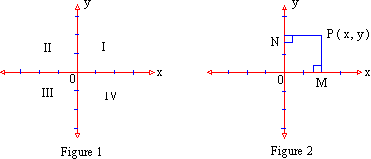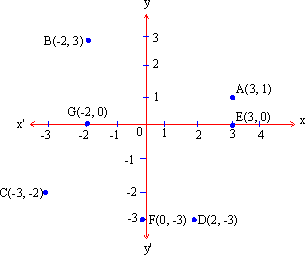Home MonkeyNotes Printable Notes Digital Library Study Guides Study Smart Parents Tips College Planning Test Prep Fun Zone Help / FAQ How to Cite New Title Request

 CHAPTER 8 : ANALYTIC GEOMETRY 8.1 Definition Co-ordinate geometry ( Analytic geometry ) is a branch of mathematics developed by Euclid, Appollonius and Decartes. It provides a bridge between Geometry and Algebra and helps us to solve geometrical problems algebraically or analytically. Each point in a plane is assigned an ordered pair of numbers. This ordered pair of numbers represents the placement of the point relative to two mutually perpendicular and intersecting lines. In fact the two lines are number lines. They are called as co-ordinate axes. One axis is horizontal and is called the x - axis. The second one is the vertical axis and is called the y - axis. Their point of intersection is called the origin. As one can see, these two lines divide the plane into four disjoint convex sets. These sets are called quadrants. These quadrants are labeled in figure 1. Using the system of co-ordinate axis we see that every point on the plane is either on the axes or in one of the four quadrants. Now notice that on the x - axis numbers to right of the origin 0 are positive and to the left of the origin are negative. On the y - axis, numbers above the origin are positive and below the origin are negative. Also, note that the first number of the ordered pair associated with any point is called the x - co-ordinate or abscissa, while the second number is called y - co-ordinate or simply ordinate.Let P be the point associated with the ordered pair ( x, y ). See figure 2. PN, the distance of P from y - axis (with proper sign) is the x-coordinate or abscissa. PN, the distance of P from x-axis (with proper sign) is the y-coordinate or ordinate. Note that : In the Quadrant I, ( +, + ) Quadrant (x, y ) Þ In the Quadrant II ( -, + ) In the Quadrant III ( -, - ) always In the Quadrant IV ( +, - ) Example State to which quadrant or to which axis do the following points belong ? (3,4), (0,2), (-3,-5), (-7,0), (6, -3), (-2, 5), (1.5, -2.3), (-Ö3 , -Ö2 ) Note : - "Every Point on the x-axis has y-ordinate = 0" "Every point on the y-axis has x-coordinate = 0" Solution :    Points                axis or quadrant (3,4)              ®    In the Quadrant (I) (0,2)              ®    on the y - axis (-3,-5)           ®    In the Quadrant - (III) (-7,0)            ®    on the x - axis (6, -3)           ®     In the Quadrant (IV) (-2, 5)           ®     In the Quadrant (II) (1.5, -2.3)    ®      In the Quadrant (IV) (-Ö3 , -Ö2 )  ®      In the Quadrant (III)   Example Plot the following points. A(3,1), B(-2,3), C(-3,-2), D(2,-3), E(3,0), F(0, -3), G(-2,0)Figure 3 Index 8.1 Definition8.2 Relations, Graphs and Symmetry8.3 Slopes and Intercepts Chapter 9
All Contents Copyright © All rights reserved.
Further Distribution Is Strictly Prohibited.

 Search: All Products Books Popular Music Classical Music Video DVD Toys & Games Electronics Software Tools & Hardware Outdoor Living Kitchen & Housewares Camera & Photo Cell Phones Keywords: